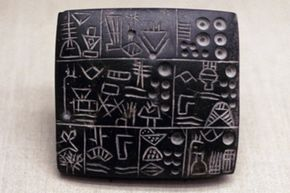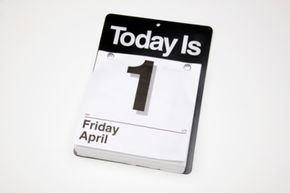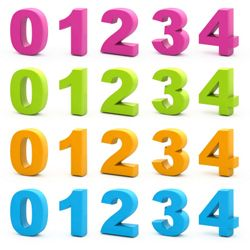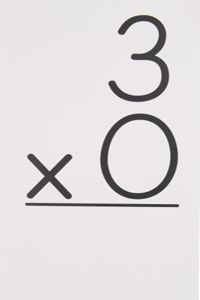# How Zero Works

By: Josh Clark

Alex the grey parrot was probably bored. He was 27 years old and for 26 of those years, he'd had researchers similar to the ones now testing him ask primitive questions like, "Alex, what color is four?" Typically, he was a whiz at these kinds of tests; he would scan the tray with a variety of objects on it, find the four orange rods and answer, "orange." In fact, in tests of his ability to count to four, he answered correctly 80 percent of the time in this particular study. But today he seemed bored. He'd been doing what the researchers called "balking" for two weeks now: staring at the ceiling, reciting a word over and over and requesting to be put back in his cage.

So one can imagine the surprise among the psychologists conducting the counting experiment with him, when after all this balking, he interjected the word "none." After testing a new hypothesis they generated following Alex's spontaneous use of the "none," the researchers found that their suspicions were correct. Alex was using "none" as a word for "zero," in this case the absence of quantity. He scored better in tests of zero quantity (83.3 percent) than he did for identifying four objects.

The fact that, when asked how many spools were present on the tray when indeed there were zero spools present, a parrot would correctly answer none was described by the researchers as "striking" [source: Pepperberg and Gordon]. After all, it was only a couple thousand years ago that zero made its first appearance among humans. It's been even less time that we've understood zero as a number.

Then again, under certain circumstances, zero isn't a number at all. In some cases, it's the opposite of infinity. In other cases, it's the mathematical equivalent of a bump on a log. In still others, it's the pivot point for all other numbers. And in any case, it is the number that captures the human imagination more than any other. The very existence of this article is evidence of that; you won't find the article How Eighteen Works anywhere on this site. Zero is also the number -- aside, arguably, from 666 -- with the darkest connotations. Cultures across the span of time and space long shunned the concept of zero, equating it with primeval void and chaos, the ingredients for the Christian concept of Hell.

In this article, we'll examine zero as a concept, a number and a key to mathematics. On the next page, we'll look at how humans came across it in the first place.

## Discovery of Zero as a Placeholder; AbhorrenceThe Mesopotamian city-state of Sumer hosted the first appearance of the concept of zero as placeholder in recording numbers.
Photos.com/Thinkstock

It's probably better to say that zero was discovered rather than invented. This idea is supported by the number of places across time and space that it simply appeared to humanity, only to be misunderstood, reviled or simply unused.

One of the first organized civilizations, the Mesopotamian city-state of Sumer, was probably the first to flirt with the concept of zero as a symbol of nothingness about 5,000 years ago [source: Matson]. On baked clay tablets used for mundane records like tax receipts or grain inventory, a pair of diagonal parallel lines were used to denote a place where there was no number. We take this concept of zero as a placeholder for granted today, but it was a revolutionary concept that allowed for much wider numbers to be represented using fewer characters, which in turn allowed for faster calculating.

Zero as a placeholder makes sense when you look at a string of numbers the way we use them today, as points that follow a specific order. For example, the number 3,024 can also be expressed as three in the thousands column (the first column on the left), none in the hundreds column, two in the tens column and four in the ones column. Without zero as a placeholder for that hundreds column, how would we be able to express the substantial difference between 3,024 head of cattle a farmer had and 324?

Thousands of years after the parallel lines were introduced in Sumer, zero as a placeholder, symbolizing nothingness, became more standardized in nearby Babylon around 300 B.C. with the advent of the abacus, which gives us our conception of numeral places that we use today.

The association of zero with nothingness made some civilizations uncomfortable. Traditionally, nothingness was associated with chaos and void, the very ingredients of Hell in the Christian tradition. In other traditions, nothingness connotes the state of the universe prior to the creation of humanity. As a result, some cultures -- including the Romans, who gave us Roman numerals -- disregarded the concept of zero, either as a placeholder or even a number [source: Seife].

Instead, our concept of zero as a number came from India and the Arab world that gave us the numerals we use today.

## Zero in the West and on CalendarsBecause there wasn't a Thursday, April 0 the day before, the Gregorian calendar has a built-in flaw.
Creatas Images/Thinkstock

The Western idea of zero being more than just a placeholder came from India in the fifth century A.D. It was here that zero as a number began to take shape and spread throughout the Arab world. It was Leonardo of Pisa, better known as Fibonacci, who introduced the number zero to the West. The son of a customs officer stationed in Algeria, Leonardo was tutored by Arabs, who taught him mathematics based on the Arabic numerals we use today, including zero. Fibonacci used what he learned and wrote a book in Latin on the use of zero and the Hindu-Arabic numeral system for the West in 1202. [source: O'Connor and Robertson]. Eventually, this idea took root and became the standard counting system we use today.

Interestingly, zero also developed simultaneously and independently of its discovery in India among the Maya of Central America. To the Mayans, zero was the base to begin counting accurately, and this was reflected in the Mayan calendars. The first day of the month was zero, followed by 1 and so on.

This concept makes for much more accurate counting, especially in tracking dates and in fact makes the Mayan calendar technically superior to the one we use today. The Gregorian calendar that is predominant in the West is based on the Roman form of counting, which did not include zero in any form (despite being introduced about 400 years after Fibonacci's book). As a result, there is no year 0 A.D. or 0 B.C. on the Gregorian calendar. By skipping over zero when dating the years following B.C., a small but noteworthy mathematics time bomb is set. Because there is no zero counted, new decades, centuries and millennia actually begin a year following their normally celebrated date. For example, the new millennium didn't actually begin until January 1, 2001, despite being celebrated by the Western world on January 1, 2000.

This misunderstanding arises from the fact that there since there is no zero year, a decade doesn't end after the ninth year, as it should. Instead, counting begins at 1, which means the tens column is reached before the transition to a new decade (or hundreds column for century and so on). Beginning a count from zero is at the heart of zero as a number, though it can seem foreign to Westerners. If you're having trouble conceiving of this, just remember that there are 10 single-digit numbers, zero through nine. Anything after that falls in the tens place or higher. But what about what's below zero? It's here that we begin to reach zero's rightful place in mathematics.

## Zero's Rightful Place in NumberingZero is a whole number (an integer) and while it's neither a negative or a positive number, it is both a non-negative and a non-positive number. Cool.

To get around the imprecision of leaving zero out of calendars, Western astronomers (who use dating frequently in their work), devised a system in the late 17th and early 18th centuries that designated the Gregorian calendar year 1 B.C. as year 0 [source: Stockton]. From there, the years follow the standard for counting with zero, adding a plus sign ahead of A.D. years and a minus sign before those designated B.C. This follows the rightful place of zero in the infinite galaxy of numbers.

As a number, zero has a specific place, precisely between one and negative one. On either side of zero, numbers stretch on infinitely. This makes zero the only number that is neither negative nor positive. It manages to straddle the line; it's counted as an integer (a whole number), a non-negative number and a non-positive number, yet it's also not included in positive or negative numbers [source: Wolfram].

Interestingly, while zero is a whole number, its existence also allows us to break numbers up into ratios, or fractions that can be expressed using the decimal system. Prior to the decimal system, which allows fraction of a whole number to be expressed with a zero followed by a decimal point and then the fraction of the number, ratios could be difficult, especially extensive ones. The decimal system converts fractions of whole numbers into arrangements similar to large whole numbers, with numerical places representing not tens hundreds or thousands, but tenths, hundredths and thousandths. In much the same way that whole numbers pivot between negative and positive on zero, decimals extend out infinitely from zero, since a whole number is expressed as 1.0, and a decimal of that is 1.947; everything to the right of the decimal is less than 1 and is, in a sense, encapsulated within the zero.

The advent of the decimal system made possible by zero allowed for the great precision required to carry out math and science. For example, a universe without zero makes Pi, which describes the circumference of circles, impossible. This supports the notion that zero wasn't "invented" by humans; it was discovered.

## Zero's Strange PropertiesThe multiplication property of zero: Regardless of what the other number is, multiplying by zero always results in an answer of zero.
Getty Images/Thinkstock

That zero manages to be both a non-negative and non-positive integer yet is neither negative nor positive is just one of the unique properties of the number. In fact, there is a group of these strange characteristics called the properties of zero.

The addition property of zero says that if you add or subtract zero from any other number, the answer will always result in the other number. 5+0=5 and 9,000,017-0=9,000,017, for example. It reflects the concept of zero as representing nothing -- so nothing added to something leaves that something unchanged -- zero is the only number that doesn't alter other numbers through addition or subtraction.

The additive inverse property of zero reflects its position as the fulcrum between the negative and positive integers. Any two numbers whose sum is zero are additive inverses of one another. For example, if you add -5 to 5, you arrive at zero. So -5 and 5 are additive inverses of one another.

The multiplication property states what every third-grader knows: Multiplying any number by zero results in a total of zero. It's obvious once ingrained but perhaps the reason is overlooked. Multiplication is, in one effect, a shortcut for addition. 3x2 is the same as 2+2+2, so the idea that a number can be added zero times or that zero can be added to itself any number of times is mathematically senseless [source: Carasco].

The concept of dividing by zero is even more senseless, so much so there is no property for it; the concept simply doesn't exist since it can't be carried out. Even mathematicians often struggle to explain why dividing by zero doesn't work. The reason is essentially related to the multiplication property. When dividing a number by another number, for example 6/2, the result (in this case, 3) can be meaningfully plugged into a formula where the answer multiplied by the divisor equals the dividend. In other words, 6/2=3 and 3x2=6. This doesn't work with zero when we replace 2 with it as the divisor; 3x0=0, not 6 [source: Utah Math]. The concept of dividing by zero is fraught with illogical consequences, so much so that its mythical destructive power has become a joke on the Internet.

There is also the property of the zero exponent; because of the existence of negative exponents, numbers to the negative power, numbers to the zero power always equal one. Although this works mathematically, it too presents logical problems. Chiefly, zero to the zero power still equals one, although zero added or subtracted to or multiplied by itself should equal zero [source: Stapel].

Behold, the power of zero.

### Sources

• Arsham, Hossein. "Zero in four dimensions." University of Baltimore. Accessed April 18, 2011. http://home.ubalt.edu/ntsbarsh/zero/zero.htm
• Ask Dr. Math. "Division by zero." The Math Forum at Drexel University. Accessed April 5, 2011. http://mathforum.org/dr.math/faq/faq.divideby0.html
• Carasco, Jester. "Properties of zero." Basic-Mathematics.com. Accessed April 18, 2011. http://www.basic-mathematics.com/properties-of-zero.html
• Forex Realm. "Fibonacci biography and facts." Accessed April 15, 2010. http://www.forexrealm.com/technical-analysis/fibonacci/fibonacci-biography-history-facts.html
• Gray, Jeremy. "Book review: Zero: the biography of a dangerous idea." Notices of the AMS. October 200. http://www.ams.org/notices/200009/rev-gray.pdf
• Knott, Dr. Ron. "Who was Fibonacci?" University of Surrey. March 11, 1998. http://www.maths.surrey.ac.uk/hosted-sites/R.Knott/Fibonacci/fibBio.html
• Matson, John. "The origin of zero." Scientific American. August 21, 2009. http://www.scientificamerican.com/article.cfm?id=history-of-zero
• O'Connor, J.J. and Robertson, E.F. "The history of zero." University of St. Andrews. November 2000. http://www-history.mcs.st-and.ac.uk/HistTopics/Zero.html
• Pepperberg, Irene and Gordon, Jesse D. "Number comprehension by a grey parrot (Psittacus erithacus), including a zero-like concept." Journal of Comparative Psychology. 2005. http://www.alexfoundation.org/papers/JCPAlexComp.pdf
• Saudi Aramco World. "Zero, key to numbers." November 1961. http://www.saudiaramcoworld.com/issue/196109/zero.key.to.numbers.htm
• Seife, Charles. "Zero: the biography of a dangerous idea." Penguin. 2000. http://books.google.com/books?id=obJ70nxVYFUC
• Singh, Simon. "5 numbers - zero." BBC. March 11, 2002. http://www.bbc.co.uk/radio4/science/5numbers1.shtml
• Stapel, Elizabeth. "Negative exponents." Purplemath. Accessed April 18, 2011. http://www.purplemath.com/modules/exponent2.htm
• Stockton, J.K. "Date miscellany I." March 10, 2010. http://www.merlyn.demon.co.uk/miscdate.htm#AstDat
• Teresi, Dick. "Zero." The Atlantic. July 1997. http://www.theatlantic.com/past/docs/issues/97jul/zero.htm
• The Straight Dope. "Is zero a number?" Accessed April 5, 2011.http://www.straightdope.com/columns/read/1633/is-zero-a-number
• University of Utah Mathematics Department. "Why can't we divide by zero?" February 17, 1997. http://www.math.utah.edu/~pa/math/0by0.html
• Wolfram Math World. "Natural number." Accessed April 5, 2011. http://mathworld.wolfram.com/NaturalNumber.html